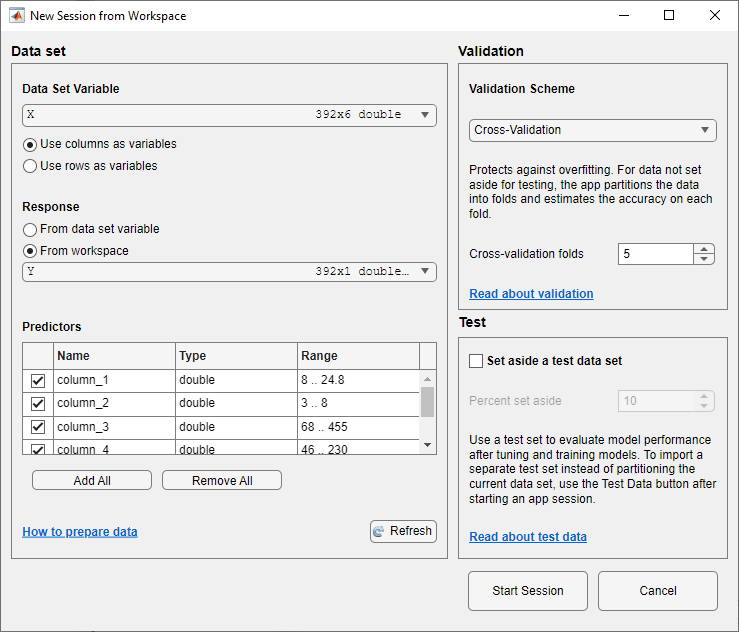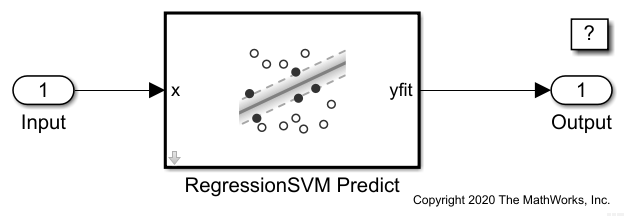# Predict Responses Using RegressionSVM Predict Block

This example shows how to train a support vector machine (SVM) regression model using the Regression Learner app, and then use the RegressionSVM Predict block for response prediction in Simulink®. The block accepts an observation (predictor data) and returns the predicted response for the observation using the trained SVM regression model.

### Train Regression Model in Regression Learner App

Train an SVM regression model by using hyperparameter optimization in the Regression Learner App.

1. In the MATLAB® Command Window, load the `carbig` data set, and create a matrix containing most of the predictor variables and a vector of the response variable.

```load carbig X = [Acceleration,Cylinders,Displacement,Horsepower,Model_Year,Weight]; Y = MPG;```

2. Open Regression Learner. On the Apps tab, in the Apps section, click the Show more arrow to display the apps gallery. In the Machine Learning and Deep Learning group, click Regression Learner.

3. On the Regression Learner tab, in the File section, select New Session and select From Workspace.

4. In the New Session from Workspace dialog box, select the matrix `X` from the Data Set Variable list. Under Response, click the From workspace option button and select the vector Y from the workspace. The default validation option is 5-fold cross-validation, to protect against overfitting. For this example, do not change the default settings.5. To accept the default options and continue, click Start Session.

6. Select an optimizable SVM model to train. On the Regression Learner tab, in the Models section, click the Show more arrow to open the gallery. In the Support Vector Machines group, click Optimizable SVM.7. In the Train section, click Train All and select Train Selected. The app displays a Minimum MSE Plot as it runs the optimization process. At each iteration, the app tries a different combination of hyperparameter values and updates the plot with the minimum validation mean squared error (MSE) observed up to that iteration, indicated in dark blue. When the app completes the optimization process, it selects the set of optimized hyperparameters, indicated by a red square. For more information, see Minimum MSE Plot.

The app lists the optimized hyperparameters in both the Optimization Results section to the right of the plot and the Model Hyperparameters section of the model Summary tab. In general, the optimization results are not reproducible.

8. Export the model to the MATLAB workspace. On the Regression Learner tab, in the Export section, click Export Model and select Export Model, then click OK. The default name for the exported model is `trainedModel`.

Alternatively, you can generate MATLAB code that trains a regression model with the same settings used to train the SVM model in the app. On the Regression Learner tab, in the Export section, click Generate Function. The app generates code from your session and displays the file in the MATLAB Editor. The file defines a function that accepts predictor and response variables, trains a regression model, and performs cross-validation. Change the function name to `trainRegressionSVMModel` and save the function file. Train an SVM model by using the `trainRegressionSVMModel` function.

`trainedModel = trainRegressionSVMModel(X,Y);`

9. Extract the trained SVM model from the `trainedModel` variable. `trainedModel` contains a `RegressionSVM` model object in the `RegressionSVM` field.

`svmMdl = trainedModel.RegressionSVM;`

Because hyperparameter optimization can lead to an overfitted model, the recommended approach is to create a separate test set before importing your data into the Regression Learner app and see how the optimized model performs on your test set. For more details, see Train Regression Model Using Hyperparameter Optimization in Regression Learner App.

This example provides the Simulink model `slexCarDataRegressionSVMPredictExample.slx`, which includes the RegressionSVM Predict block. You can open the Simulink model or create a new model as described in this section.

Open the Simulink model `slexCarDataRegressionSVMPredictExample.slx`.

```SimMdlName = 'slexCarDataRegressionSVMPredictExample'; open_system(SimMdlName)```The `PreLoadFcn` callback function of `slexCarDataRegressionSVMPredictExample` includes code to load the sample data, train the SVM model, and create an input signal for the Simulink model. If you open the Simulink model, then the software runs the code in `PreLoadFcn` before loading the Simulink model. To view the callback function, in the Setup section on the Modeling tab, click Model Settings and select Model Properties. Then, on the Callbacks tab, select the `PreLoadFcn` callback function in the Model callbacks pane.

To create a new Simulink model, open the Blank Model template and add the RegressionSVM Predict block. Add the Inport and Outport blocks and connect them to the RegressionSVM Predict block.

Double-click the RegressionSVM Predict block to open the Block Parameters dialog box. You can specify the name of a workspace variable that contains the trained SVM model. The default variable name is `svmMdl`. Click the Refresh button. The dialog box displays the options used to train the SVM model `svmMdl` under Trained Machine Learning Model.The RegressionSVM Predict block expects an observation containing 6 predictor values. Double-click the Inport block, and set the Port dimensions to 6 on the Signal Attributes tab.

Create an input signal in the form of a structure array for the Simulink model. The structure array must contain these fields:

• `time` — The points in time at which the observations enter the model. The orientation must correspond to the observations in the predictor data. So, in this example, `time` must be a column vector.

• `signals` — A 1-by-1 structure array describing the input data and containing the fields `values` and `dimensions`, where `values` is a matrix of predictor data, and `dimensions` is the number of predictor variables.

Create an appropriate structure array for the `slexCarDataRegressionSVMPredictExample` model from the `carsmall` data set.

```load carsmall testX = [Acceleration,Cylinders,Displacement,Horsepower,Model_Year,Weight]; testX = rmmissing(testX); carsmallInput.time = (0:size(testX,1)-1)'; carsmallInput.signals(1).values = testX; carsmallInput.signals(1).dimensions = size(testX,2);```

To import signal data from the workspace:

• Open the Configuration Parameters dialog box. On the Modeling tab, click Model Settings.

• In the Data Import/Export pane, select the Input check box and enter `carsmallInput` in the adjacent text box.

• In the Solver pane, under Simulation time, set Stop time to `carsmallInput.time(end)`. Under Solver selection, set Type to `Fixed-step`, and set Solver to `discrete (no continuous states)`.

`sim(SimMdlName);`